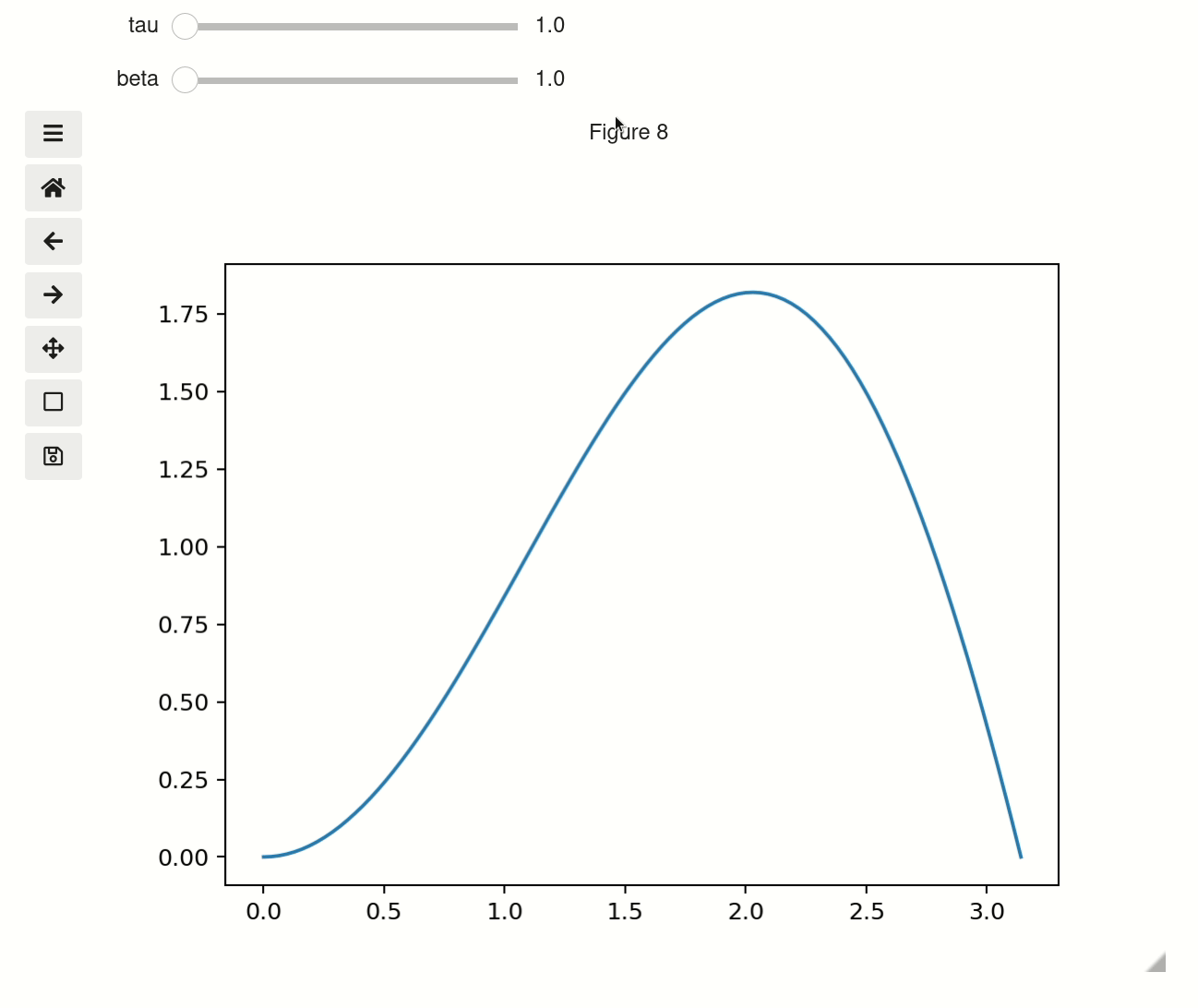# Plot¶

The interactive plot function is availiable either as mpl_interactions.pyplot.interactive_plot or as mpl_interactions.ipyplot.plot. It will behave equivalently to the normal matplotlib pyplot.plot function if you don’t pass it any non-plotting kwargs. If you do pass in non-plotting kwargs and one or both of x or y is a function then the kwargs will be converted into widgets to control the function.

[ ]:

%matplotlib ipympl
import ipywidgets as widgets
import matplotlib.pyplot as plt
import numpy as np

import mpl_interactions.ipyplot as iplt

[ ]:

x = np.linspace(0, np.pi, 100)
tau = np.linspace(1, 10, 100)
beta = np.linspace(0.001, 1)

def f(x, tau, beta):
return np.sin(x * tau) * x ** beta

fig, ax = plt.subplots()
controls = iplt.plot(x, f, tau=tau, beta=beta)## Troubleshooting¶

If instead of a plot you got an output that looks like this: VBox([IntSlider(min=0, max=10 ..... and you are using jupyterlab then you probably need to install jupyterlab-manager:

conda install -c conda-forge nodejs=12
jupyter labextension install @jupyter-widgets/jupyterlab-manager


after the install and finishes refresh the browser page and it should work

## set parameters with tuples¶

When you use tuples with length of 2 or 3 as a parameter then it will be treated as an argument to linspace. So the below example is equivalent to first example

[ ]:

fig2, ax2 = plt.subplots()
controls2 = iplt.plot(x, f, tau=(1, 10, 100), beta=(1, 10))## Use sets for categorical values¶

sets with three or fewer items will be rendered as checkboxs, while with more they will use the selection widget. Unfortunately sets are instrinsically disordered so if you use a set you cannot garuntee the order of the categoricals. To get around this if you have a set of single tuple it will be ordered. i.e. {('sin', 'cos')} will show up in the order: sin, cos. While {'sin', 'cos'} will show up in the order: cos, sin

[ ]:

def f(x, tau, beta, type_):
if type_ == "sin":
return np.sin(x * tau) * x ** beta
elif type_ == "cos":
return np.cos(x * tau) * x ** beta
elif type_ == "beep":
return x * beta / tau
else:
return x * beta * tau

fig3, ax3 = plt.subplots()
controls3 = iplt.plot(
x, f, tau=(0.5, 10, 100), beta=(2, 10), type_={("sin", "cos", "beep", "boop")}
)### Using widgets for as parameters¶

You can also pass an ipywidgets widget that has a value attribute

[ ]:

def f(x, tau, beta, type_):
if type_ == "sin":
return np.sin(x * tau) * x ** beta
elif type_ == "cos":
return np.cos(x * tau) * x ** beta

tau = widgets.FloatText(value=7, step=0.1)
fig4, ax4 = plt.subplots()
controls4 = iplt.plot(x, f, tau=tau, beta=(1, 10), type_={("sin", "cos")})


### With multiple functions¶

You have multiple interactive functions by passing the controls object to subsequent calls.

[ ]:

x = np.linspace(0, np.pi, 100)
tau = np.linspace(0.5, 10, 100)
beta = np.linspace(1, 10, 100)

def f1(x, tau, beta):
return np.sin(x * tau) * x * beta

def f2(x, tau, beta):
return np.sin(x * beta) * x * tau

fig, ax = plt.subplots()
controls = iplt.plot(x, f1, tau=tau, beta=beta, label="f1")
iplt.plot(x, f2, label="f2", controls=controls)
_ = plt.legend()


### Styling of plot¶

You can either use the figure and axis objects returned by the function, or if the figure is the current active figure the standard plt.__ commands should work as expected. You can also provide explict plot_kwargs to the plt.plot command that is used internally using the plot_kwargs argument

You can control how xlim/ylims behave using the x_scale/y_scale arguments. The options are: 1. stretch - never shrink the x/y axis but will expand it to fit larger values 2. auto - autoscale the x/y axis for every plot update 3. fixed - always used the initial values of the limits 4. a tuple - You can pass a value such as [-4,5] to have the limits not be updated by moving the sliders.

## Title¶

You can make the title auto update with information about the values by using the title argument. Just use the name of one of the parameters as in a format specifier in the string. e.g. to put the value of tau in and round it to two decimals use the following title string: {'tau:.2f}'

[ ]:

x_ = np.linspace(0, np.pi, 100)
tau = np.linspace(1, 10, 100)

def f_x(tau):
return x_

def f_y(x, tau):
return np.sin(x * tau) * x

fig, ax = plt.subplots()
controls = iplt.plot(
f_x,
f_y,
tau=tau,
xlim="stretch",
ylim="auto",
title="the value of tau is: {tau:.2f}",
label="interactive!",
)

# you can still use plt commands if this is the active figure
plt.ylabel("yikes a ylabel!")

# you can new lines - though they won't be updated interactively.
plt.plot(x, np.sin(x), label="Added after, not interactive")

_ = plt.legend()  # _ to capture the annoying output that would otherwise appear


## Slider precision¶

You can change the precision of individual slider displays by passing slider_format_string as a dictionary. In the below cell we give tau 99 decimal points of precision and use scientific notation to display it. The other sliders will use the default 1 decimal point of precision.

[ ]:

x = np.linspace(0, np.pi, 100)
tau = np.linspace(0.5, 10, 100)
beta = np.linspace(1, 10, 100)

def f1(x, tau, beta):
return np.sin(x * tau) * x * beta

def f2(x, tau, beta):
return np.sin(x * beta) * x * tau

fig, ax = plt.subplots()
controls = iplt.plot(x, f1, tau=tau, beta=beta, slider_formats={"tau": "{:.50e}"})


## fixed y-scale¶

You can also set yscale to anything the matplotlib will accept as a ylim

[ ]:

x = np.linspace(0, np.pi, 100)
tau = np.linspace(1, 10, 100)

def f(x, tau):
return np.sin(x * tau) * x ** tau

fig, ax = plt.subplots()
iplt.plot(x, f, tau=tau, ylim=[-3, 4], label="interactive!")

# you can still use plt commands if this is the active figure
plt.ylabel("yikes a ylabel!")
plt.title("Fixed ylim")

# you can new lines - though they won't be updated interactively.
plt.plot(x, np.sin(x), label="Added after, not interactive")

_ = plt.legend()  # _ to capture the annoying output that would otherwise appear

[ ]: